Courses

# Dipole in Uniform & Non-Uniform Electric Field Class 12 Notes | EduRev

## Class 12 : Dipole in Uniform & Non-Uniform Electric Field Class 12 Notes | EduRev

The document Dipole in Uniform & Non-Uniform Electric Field Class 12 Notes | EduRev is a part of the Class 12 Course Physics Class 12.
All you need of Class 12 at this link: Class 12

INTRODUCTION TO DIPOLE IN UNIFORM EXTERNAL FIELD
If a dipole is kept in an external electric field, it experiences a rotating effect. By external electric field, we mean electric field that is not induced by dipole itself. The rotating effect is also called torque on the dipole. How we can calculate the torque on a dipole and what are its applications? This can be done by calculating the net torque on opposite charges of the dipole.

Dipole in Uniform External Field
To find torque on a dipole from an external field, consider there is electric dipole placed in an uniform external field. The uniform external electric field is produced externally and is not induced by dipole.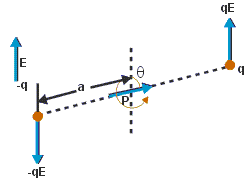Fig: An electric dipole placed in non-uniform external electric field

The external electric field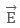will produce electric force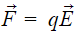on positive charge in upward direction (same direction as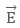and on negative charge in downward direction (opposite direction to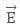).
We can see that the dipole is in transitional equilibrium as net force on the dipole is zero.
What about the rotational equilibrium? Is it also zero? If that was the case, then the dipole would have been stationary in position, but experimentally it is found that the dipole rotates with some angular velocity.
This is because, both the electrostatic force that is,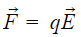acts a torque in a clockwise direction, thereby making the dipole to rotate in a uniform external electric field.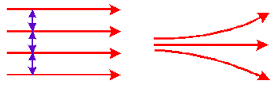Fig: Uniform and Non-uniform electric field

Torque always acts in a couple, and its magnitude equals to the product of force and its arm. Arm is the distance between the point where the force acts and the point which rotates the dipole. In the dipole placed in the uniform external electric field, we take origin as the point. Torque is denoted by the symbol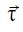and as it has a direction, it is a vector quantity.

Mathematically,
Magnitude of torque = q E × 2a sin θ
τ = 2 q a E sin θ
Since, the dipole moment (p = 2qa)
τ = p E sin θ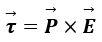The vector form of torque is the cross product of dipole moment and electric field.
To understand what cross product is, let’s take an example.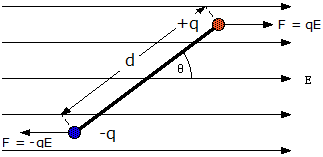Fig: Torque rotates the dipole in uniform electric field

The net external force acting on the dipole will be zero, hence it will not translate in space. But the same cannot be said about the net torque on the dipole.
In short,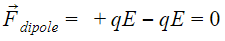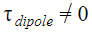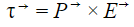Where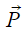represents the dipole moment of the electric dipole and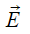represents the external electric field in which the dipole is kept.

Observations in net force and torque
Taking the nature of electric field and position of the dipole, following remarks will come out:

• If the dipole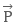and external electric field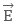are parallel, that is, angle between them is zero, then the dipole will feel zero torque. That is, no rotational effect.
• If the external electric fieldis non-uniform, then net force on the dipole ≠ 0, and torque will create rotation. Hence, it would be a combined rotational and translational motion.
• If the dipole external electric fieldare antiparallel, that is, angle between them is non-zero, then the dipole will feel zero torque.
• When the electric dipoleand electric fieldare parallel, the direction of net force will be in direction of increasing electric field.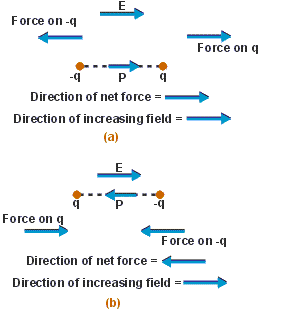Fig: Direction of net force depends on orientation of electric dipole
• When the electric dipoleand electric fieldare anti-parallel, then the direction of net force will be in direction of decreasing electric field.
• Force and Torque on a dipole placed in a uniform external fieldvaries with the orientation of dipole in free space

PHYSICAL SIGNIFICANCE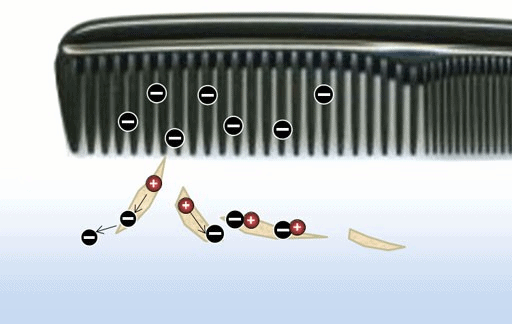Fig: Comb attract dry paper piece

When we comb our dry hair and bring it near to some paper pieces, we find that the comb attracts the paper pieces. The comb gains charge, from our hair by the process of rubbing and induce a charge in the uncharged paper. In another way, the comb polarizes the pieces of paper that is, generate a net dipole moment in the direction of electric field. Also, since the electric field is non-uniform, the paper pieces move in the direction of the comb.

Offer running on EduRev: Apply code STAYHOME200 to get INR 200 off on our premium plan EduRev Infinity!

## Physics Class 12

132 videos|260 docs|126 tests

,

,

,

,

,

,

,

,

,

,

,

,

,

,

,

,

,

,

,

,

,

;